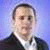Dec 2 2008   5:26PM GMT

# Crawford’s Mainframe Assembler Quiz, answers and part 2Profile: Matt Stansberry

In his October mainframe column, columnist Robert Crawford asked what commonly used Assembler instruction can be used to add three numbers together and place the answer in a fourth location. The answer is Load Address (LA).

Below is Crawford’s answer and the next Assembler Quiz.

Most of us have seen LA used to get the address of a label:

`LA R13,SAVEAREA`

But if you look in the Principle of Operations manual you will see the actual format of the instruction is as follows:

`LA R1,O(X,B)`

Where: `R1` is the register to receive the address
`X` is the index register
`B` is the base register
`O` is an offset to be added to the sum of the base and index registers

In other words, the magical thing we call an address is actually just a number computed by adding two registers together with an offset. So, at the end of this instruction sequence:
```LA R1,4 R1=4 LA R2,3 R2=3 LA R3,2(R1,R2) R3=2+4+3```

Register 3 contains 9